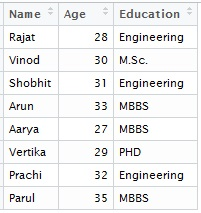## Pages

###R Tutorial 6.0

Appending is adding dataset one below other or making a vertical stack of data.

Let's see how appending is performed in R.

### How appending is different from merging ?Appending is generally used when various pieces of same information has to be collated together. e.g. Monthly Sales data, monthly premium data etc.

Merging on the other hand is used when different information pieces for the same entity are to be collated together. It requires a primary common key in the datasets to be merged.

We have already covered merging and appending inn SAS in one of our previous articles. Merging in R has also been covered. Let's now learn appending in R

### Appending in R

#Let's first create two data with list of speakers at a conference :List_1

List_1 = data.frame(Name = c("Rajat","Vinod","Shobhit","Arun"), Age = c(28,30,31,33), Education = c("Engineering","M.Sc.","Engineering","MBBS"))List_2

List_2 = data.frame( Age = c(27,29,32,35), Name = c("Aarya","Vertika","Prachi","Parul"), Education = c("MBBS","PHD","Engineering","MBBS"))Append_1

# Let's now append the lists - simply with rbind function

Append_1 = rbind(List_1,List_2)

The above example was very basic one as both were having same columns so rbind function simply placed one data below other.

Important point : The order of the variables in the data is not mandatory to be same for appending. Resultant dataset, though, maintains the first data's column order.

### Complication 1 : Inconsistent column names

# Suppose in the below example, B and C are same columns with different names

Data_x = data.frame(A = 1:5, B = 6:10)

Data_y = data.frame(A = 11:15, C = 16:20)

# When we try to append these directly, R throws an error

Append_xy = rbind(Data_x, Data_y)

We get an error n console:
Error in match.names(clabs, names(xi)) :   names do not match previous names

# In such cases, we can rename the column in one of the datasets to enable appending

names(Data_y) = "B"
Append_xy = rbind(Data_x, Data_y)

Voila ! it works now .

### Complication 2 : Different columns in datasets

We have one data with 3 coulmns and second one with only 2. 2 columns however are same.

Example :

List_A = data.frame(Name = c("Rajat","Vinod","Shobhit","Arun"), Age = c(28,30,31,33), Education = c("Engineering","M.Sc.","Engineering","MBBS"))

List_B = data.frame( Name = c("Aarya","Vertika","Prachi","Parul"),Education = c("Engineering","M.Sc.","Engineering","MBBS"))

# In List B, Age column in missing. Let's try appending these

Append_comp = rbind(List_A,List_B)

We get an error n console:
Error in rbind(deparse.level, ...) :   numbers of columns of arguments do not match

# In such case, we match the number of column by populating NULL column in data

# Try this and it would work fine

List_B\$Age = NA

Append_comp = rbind(List_A,List_B)

Enjoy reading our other articles and stay tuned with us.

Kindly do provide your feedback in the 'Comments' Section and share as much as possible.